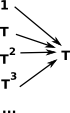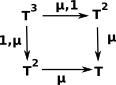# Maths - Category Theory - Algebra

There are at least two approaches to algebra in category theory:

• Lawvere algebraic theories

The relationship between algebras and monads is discussed on the page here. On the remainder of this page we discuss Lawvere algebraic theories.

## Lawvere Algebraic TheoriesIn this approach, instead of using signatures as we would in universal algebra, we define arrows from powers of our structure 'T'. This means that we can only define algebras on a structure with associative products.
 We can then use this to define the axioms like this:## Models of Algebraic Theories

See page here for a general discussion of algebraic theories and models of those theories.

A model of the theory 'T' is a functor F: T->Set which preserves finite products.

## Category Theory and W-types

W-types are initial algebras for polynomial endofunctors.

x

Where I can, I have put links to Amazon for books that are relevant to the subject, click on the appropriate country flag to get more details of the book or to buy it from them.The Princeton Companion to Mathematics - This is a big book that attempts to give a wide overview of the whole of mathematics, inevitably there are many things missing, but it gives a good insight into the history, concepts, branches, theorems and wider perspective of mathematics. It is well written and, if you are interested in maths, this is the type of book where you can open a page at random and find something interesting to read. To some extent it can be used as a reference book, although it doesn't have tables of formula for trig functions and so on, but where it is most useful is when you want to read about various topics to find out which topics are interesting and relevant to you.